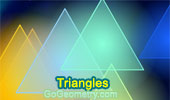# Triangles: Theorems and Problems 2Second Fermat Point: Static diagram.
Second Fermat Point: Dynamic diagram.
Triangle, Equilateral triangles, Concurrent lines, GeoGebra, HTML5 Animation for Tablets (iPad, Nexus)

Kosnita's Theorem.
Circumcenters, Concurrent lines.

Routh's Theorem - Index
Triangle, Cevians, Area, Ratio.

Routh's Theorem 1.
Triangle, Cevians, Ratio of Areas.

Routh's Theorem 2.
Triangle, Cevians, Ratio of Areas.

Routh's Theorem 3.
Triangle, Cevians, Ratio of Areas.

Routh's Theorem 4.
Triangle, Cevians, Ratio of Areas.

Euclid's Elements Book I, Proposition 7
Given two straight lines constructed on a straight line (from its extremities) and meeting in a point,...

iPad Apps, Geometry Designer Illustration. Circle of van Lamoen.
Interactive Geometry Software for iPad, iPhone, iPod.

Go to Page: Previous | 1 | 2 | 3 | 4 | 5 | 6 | 7 | 8 | 9 | 10 | 11 | 12 | 13 | 14 | 15 | 16 | 17 | 18 | 19 | 20 | 21 | 22 | 23 | 24 | 25 | 26 | 27 | 28 | 29 | 30 | 40 | Next

 Home | Search | Geometry | Polygon | Email